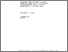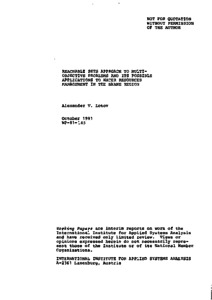Reachable Sets Approach to Multiobjective Problems and its Possible Applications to Water Resources Management in the Skane Region

Lotov, A.V. (1981). Reachable Sets Approach to Multiobjective Problems and its Possible Applications to Water Resources Management in the Skane Region. IIASA Working Paper. IIASA, Laxenburg, Austria: WP-81-145Preview
Text
WP-81-145.pdf

Abstract

Decision making in a lot of resources supply and resources allocation problems is related to sophisticated multiobjective analysis. The concept of a man-computer simulation system was suggested as a tool for decision making in problems of this kind, especially in the case of water resources (Moiseev et al. 1980). Within the framework of such a system the analyst and the expert employ a full range of operational research methods (simulation, optimization, multiobjective, informal and game-theoretical ones) to address multiobjective problems by means of the hierarchical system of mathematical models of the system under study. Various forms of mathematical models can be studied by means of simulation experiments. To establish control variables (to formulate scenarios) in a simulation study the expert may use optimization techniques applied to models simpler than the simulation ones. It is reasonable to study the problem of criteria formulation in optimization problems (the objectives convolution problem) by means of multiobjective techniques and simple (screening) models. The multiobjective study is the most important part of investigation based on the simulation system, because it is the multiobjective investigation that gives a general understanding of the system under study.

This paper treats a new approach to multiobjective problems investigation. This approach is called the Generalized Reachable Sets (GRS) approach and belongs to generating multiobjective methods (Cohon 1978). It employs an explicit representation of a set of all reachable objective values. In contrast to different generating multiobjective methods, the mathematical background of the GRS approach is the linear inequalities techniques.

This approach is used now at the Computing Center of the USSR Academy of Sciences in various tasks.

The structure of the paper is as follows: first the mathematical background of the approach is outlined, and then possible applications of the approach to the Skane water resources management are discussed.

Item Type: Monograph (IIASA Working Paper) System and Decision Sciences - Core (SDS) IIASA Import 15 Jan 2016 01:49 27 Aug 2021 17:10 http://pure.iiasa.ac.at/1616View Item